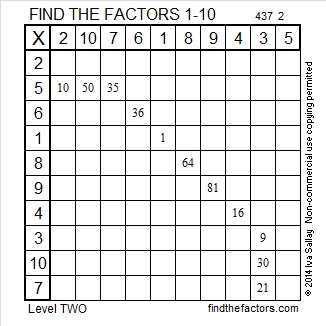# 233 and Level 2

• 233 is a prime number.
• Prime factorization: 233 is prime.
• The exponent of prime number 233 is 1. Adding 1 to that exponent we get (1 + 1) = 2. Therefore 233 has exactly 2 factors.
• Factors of 233: 1, 233
• Factor pairs: 233 = 1 x 233
• 233 has no square factors so its square root cannot be simplified. √233 ≈ 15.264How do we know that 233 is a prime number? If 233 were not a prime number, then it would be divisible by at least one prime number less than or equal to √233 ≈ 15.264. Since 233 cannot be divided evenly by 2, 3, 5, 7, 11, or 13, we know that 233 is a prime number.Print the puzzles or type the factors on this excel file: 10 Factors 2014-09-15This site uses Akismet to reduce spam. Learn how your comment data is processed.